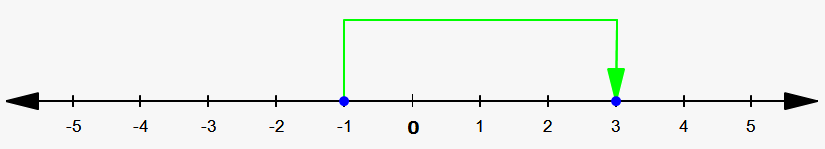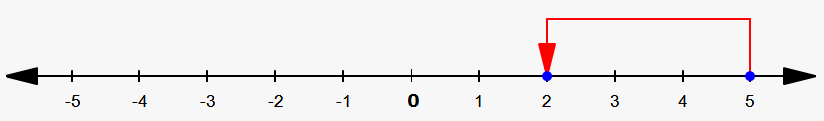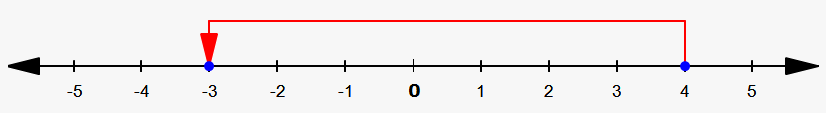# Adding Integers on a Number Line

With the help of number line we can add integers.

It is better to show on examples how to do this.

Example 1. Find -1+4.

We draw -1 on a number line. Since we add positive number (4) we move 4 units to the right. Result is 3. So, -1+4=3.Let's see how to add negative numbers.

Example 2. Find 5+(-3).

We draw 5 on a number line. Since we add negative number (-3) we move 3 units to the left. Result is 2. So, 5+(-3)=2.Now, do the following exercise to remember this topic better.

Exercise 1. Find -5+5.